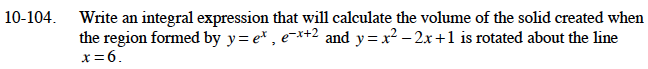### Home > CALC > Chapter 10 > Lesson 10.2.2 > Problem10-104

10-104.

Write an integral expression that will calculate the volume of the solid created when the region formed by y = ex , y = ex+2, and y = x2 − 2x + l is rotated about the line x = 6. Homework Help ✎Using the Shell Method:
The radius is 6 – x.
The height is obtained by subtracting two functions.
Use two integrals.